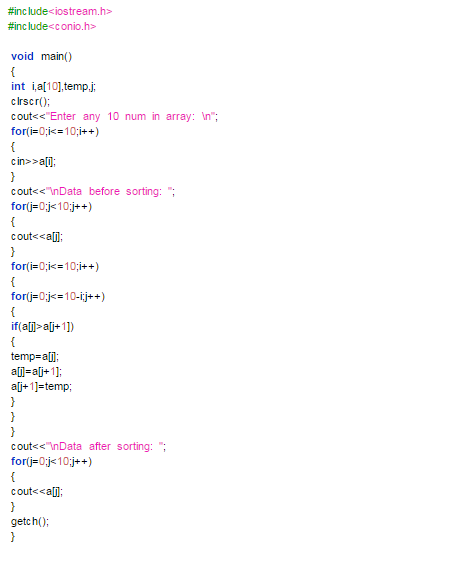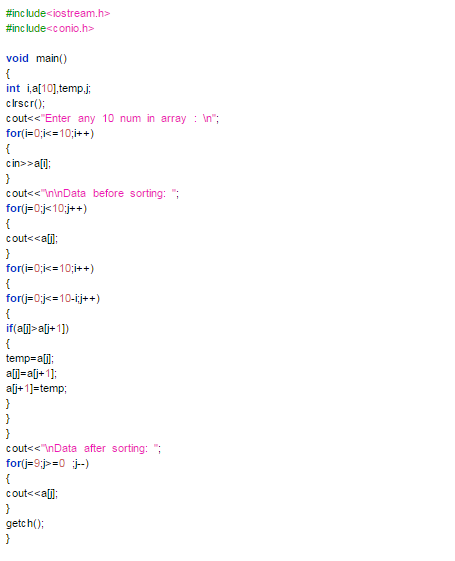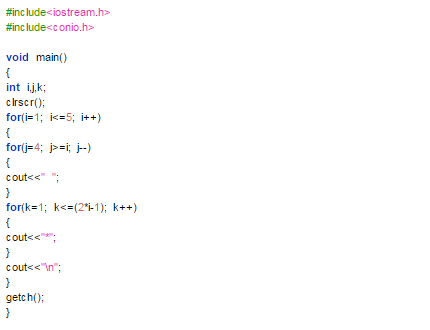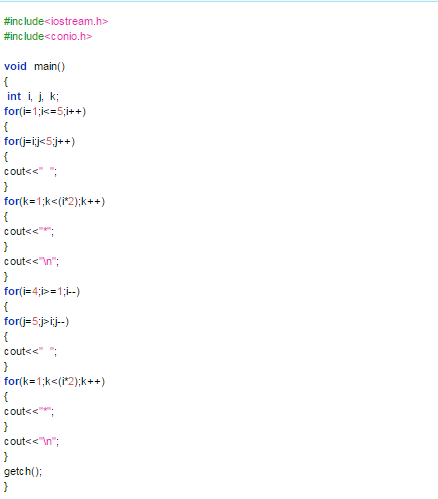# Answered! Discrete Dynamics Using a graphical representation, construct an extended state machine that is a basic counter given inputs u(t) (up…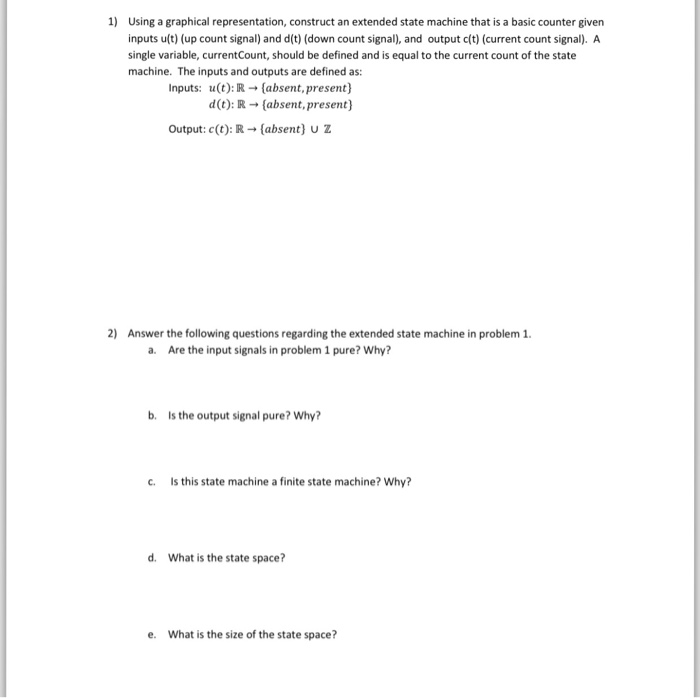Discrete Dynamics

Using a graphical representation, construct an extended state machine that is a basic counter given inputs u(t) (up count signal) and d(t) (down count signal), and output c(t) (current count signal). A single variable, current Count, should be defined and is equal to the current count of the state machine. The inputs and outputs are defined as: Inputs: u(t): R rightarrow {absent, present} d(t): R rightarrow {absent, present} Output: c(t): R rightarrow {absent} union Z Answer the following questions regarding the extended state machine in problem 1. a. Are the input signals in problem 1 pure? Why? b. Is the output signal pure? Why? c. Is this state machine a finite state machine? Why? d. What is the state space? e. What is the size of the state space?